• pandas处理多组数据的时候往往会要用到数据的合并处理,使用 concat是一种基本的合并方式.而且concat中有很多参数可以调整,合并成你想要的数据形式. 2.垂直合并（axis = 0） axis=0是预设值，因此未设定任何参数时，...
• 需求：根据某一列的值对指定的其他列做合并操作(根据A列，对BC列作合并单元格) import xlwt import pandas as pd class Merge_cell(): ''' 目标：根据某列值对指定列值进行合并 ''' def __init__(self, ...
需求：根据某一列的值对指定的其他列做合并操作(根据A列，对BC列作合并单元格)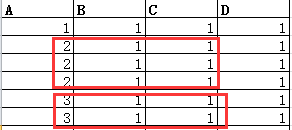import xlwt
import pandas as pd
class Merge_cell():
'''
目标：根据某列值对指定列值进行合并
'''

def __init__(self, excel_path, df, key_col, col2):
self.excel_path = excel_path
self.df = (df.drop(columns='index') if 'index' in df.columns else df)
self.key_col = key_col
self.col2 = col2
self.wb = xlwt.Workbook(excel_path)

def _get_idx(self):
groups = self.df.groupby(self.key_col)
idxs = [[groups.get_group(i).index.min() + 1, groups.get_group(i).index.max() + 1] for i in groups.size().index]
return idxs, len(idxs)

def _get_content(self, idx, key):
"""

:param idxs: 索引 [[1,2],[3,4]]
:return: 暂时保存合并单元的值
"""
import numpy as np

if not pd.isna(self.df.at[idx, key]):
temp = self.df.at[idx, key]
else:
temp = None
return temp

def merged(self):
import numpy as np
if not self.key_col:  # 如果key_cols 参数不传值，则无需合并
self.df.to_excel(self.excel_path, index=False)
return
idxs, length = self._get_idx()
line_cn = self.df.index.size
cols = list(self.df.columns.values)
column_number = {col: idx for idx, col in enumerate(cols)}
if self.key_col not in cols:  # 校验key_cols中各元素 是否都包含与对象的列
print("key_cols is not completely include object's columns")
return False
if not all([v in cols for i, v in enumerate(self.col2)]):  # 校验merge_cols中各元素 是否都包含与对象的列
print("merge_cols is not completely include object's columns")
return False
for value, i in column_number.items():  # 写表头
self.worksheet.write(0, i, value)
for key, idx in column_number.items():
if key not in self.col2:
for i in range(line_cn):
value = self.df.loc[i, key]
if not pd.isna(value):
self.worksheet.write(i + 1, idx, str(value))
else:
pass
else:
for j in idxs:
value = self._get_content(j - 1, key)
if value:
self.worksheet.write_merge(j, j, idx, idx, value)
else:
pass
self.wb.save(self.excel_path)
if __name__ == '__main__':
te = {'A': [1, 2, 2, 2, 3, 3], 'B': [1, 1, 1, 1, 1, 1], 'C': [1, 1, 1, 1, 1, 1], 'D': [1, 1, 1, 1, 1, 1]}
t_f = pd.DataFrame(te)
DF = Merge_cell('000_1.xls', t_f, 'A', ['B', 'C'])
DF.merged()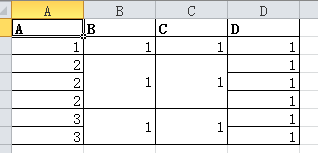参考：https://blog.csdn.net/cakecc2008/article/details/59203980


展开全文python
• 利用python的xlrd读取Excel,正确读取合并单元格数据，并打印所有单元格的数据
• pandas读取带有合并单元格的excel表格 今天在工作的时候碰到读取含有合并单元格的问题，发现读取出来的数据是这样的 发现合并单元格只有第一格有内容，其他的都被填充成空值了，找了一圈发现有一个办法可以这样解决...
pandas读取带有合并单元格的excel表格
今天在工作的时候碰到读取含有合并单元格的问题，发现读取出来的数据是这样的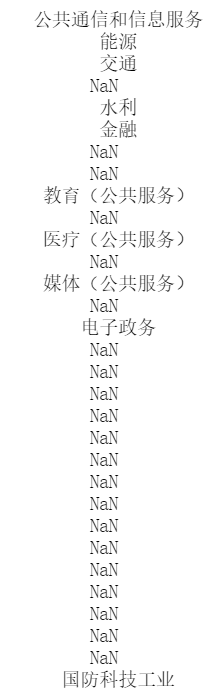发现合并单元格只有第一格有内容，其他的都被填充成空值了，找了一圈发现有一个办法可以这样解决。
df_ind['行业'] = df_ind['行业'].ffill()

pandas自带的ffill函数意思是空值是前面的值向后填充，结果如下：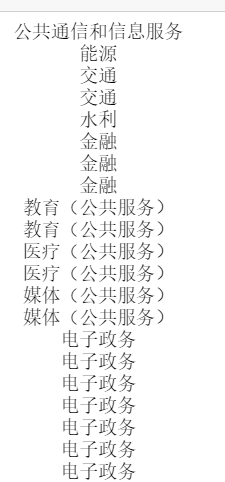展开全文数据分析
• 主要为大家详细介绍了python之DataFrame实现excel合并单元格，具有一定的参考价值，感兴趣的小伙伴们可以参考一下
• 运用pandas和openpyxl删除空行以及合并单元格 在工作中，我们有时会碰到这样一个问题，需要把一个表格的空行删除以及合并单元格，删除空行对于pandas来说小菜一碟，但是合并单元格呢，emmmmm，暂时没有想到更好的...
运用pandas和openpyxl删除空行以及合并单元格
在工作中，我们有时会碰到这样一个问题，需要把一个表格的空行删除以及合并单元格，删除空行对于pandas来说小菜一碟，但是合并单元格呢，emmmmm，暂时没有想到更好的方法。 需求如下： 我现在有这样一个表格：需要把它变成：主要步骤如下：
使用pandas对空行删除将pandas处理完的数据转成openpyxl中的Worksheet对象合并单元格和保存文件 各步骤代码如下：
pandas删除空行和填充空值
# 导入pandas和openpyxl库
import pandas as pd
from openpyxl import Workbook
from openpyxl.utils.dataframe import dataframe_to_rows
# 读取需要处理的excel文件
# 删除空行，原表替换
df.dropna(how='all',inplace=True)
# 填充空值，使用前一个值进行填充
df.fillna(axis=0,method='ffill',inplace=True)

将DataFrame数据转成openpyxl可以处理的Worksheet数据
wb=Workbook()
ws=wb.active
for row in dataframe_to_rows(df,index=False):
ws.append(row)

合并单元格和保存文件
# 获取第一列数据
type_list = []
i = 2

while True:
r = ws.cell(i, 1).value
if r:
type_list.append(r)
else:
break
i += 1

file = r'aa.xlsx'
# 判断合并单元格的始末位置
start = 0
end = 0
flag = type_list
for i in range(len(type_list)):
if type_list[i] != flag:
flag = type_list[i]
end = i - 1
if end >= start:
ws.merge_cells("A" + str(start + 2) + ":A" + str(end + 2))
start = end + 1
if i == len(type_list) - 1:
end = i
ws.merge_cells("A" + str(start + 2) + ":A" + str(end + 2))

wb.save(file)

展开全文excel python 数据分析
• pandas读取execl表格的合并单元格并填充 这是表格（三种合并单元格）： 这是填充前的输出： 填充后的输出： 代码如下：
用pandas读取execl表格的合并单元格并填充
这是表格（三种合并单元格）：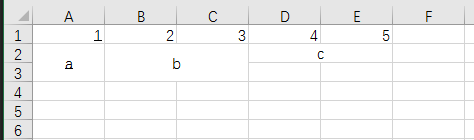这是填充前的输出：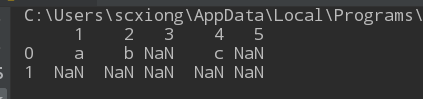填充后的输出：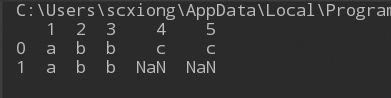代码如下：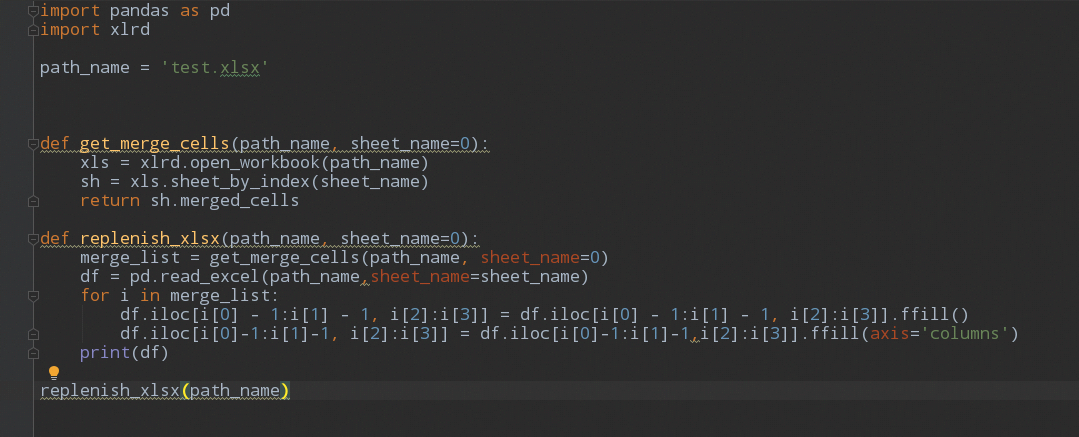展开全文• ## pandas合并表格

千次阅读 2018-12-12 10:31:25
import pandas as pd # 依次读取多个相同结构的Excel文件并创建DataFrame dfs = [] #pd.read_csv读取csv文件，如果读取Excel就用pd.read_excel for fn in ('baicheng.csv', 'baishan.csv', 'changchun.csv', 'jinlin...
• 利用pandas合并excel文件 一、多个xlsx文件合并 xlsx文件可为单sheet或多sheet混合 导入os, pandas库 import os import pandas as pd 可采用函数的形式，将文件夹作为参数，不同情况下使用时，更改参数即可 读取...python excel
• 拆分单元格是excel中经常遇到的内容之一。那么在pandas中如何实现呢？ 例如有如下数据: >>> import pandas as pd >>> import numpy as np >>> df=pd.read_excel(r'D:/myExcel/1.xlsx') &...python 数据分析 机器学习 大数据
• ## pandas --合并表格

千次阅读 2018-12-05 18:44:11
因为我经常要做数据拉取并合并存储在表格里，所以这里对我遇到的几种类型的表格合并进行讲解。 第一种就是columns是一样的，表格进行追加，如图 import glob,os from numpy import * # 下面这些变量需要您根据...
• 范围 统计 ...前3行都是合并单元格，python使用pandas读取文件后，如何处理（比如把前4行变成一行的索引？），使数据可以列索引，感谢</p>python
• 项目背景：懒 业务需求：现有各单位上报...import pandas as pd from openpyxl import load_workbook # df写入excel # 要写入的df，写入的文件名，是否写入列索引1or0 def df2xlsx(df, wenjianming, liesuoyin): wripython numpy excel
• 最近在做文本聚类时遇到，需要把某列的文本内容统一合并，其实很简单。 上代码 import pandas as pd df = pd.DataFrame({'text':['今天天气不错，适合出去玩。', '最近在上海举办了中国进口博览会，', ...
• pandas对表格进行简单的合并 ​ 无论是在学习还是平时的工作中，我们常会遇到多种信息放在不同的表里的情况，此时我们就需要将这些表格的信息合并到一起再进行操作。 首先我们建立两张学生基本信息表 ： 第一张表...python
• Pandas中不仅可以调用 .concat 函数对多个表格进行合并，还可以调用 .merge 函数进行多个表格的合并。 1 .merge合并DataFrame表格 import pandas as pd 生成多个DataFrame表格 left = pd.DataFrame({'key':['K0','...DataFrame .merge
• 使用python中的pandas，xlrd，openpyxl库完成合并excel中指定sheet的操作 # -*- coding: UTF-8 -*- import xlrd import pandas as pd from pandas import DataFrame from openpyxl import load_workbook #表格位置...
• 想自动实现xlsx合并同类项 2. 实现代码 df = pd.DataFrame(dict_w) out = io.BytesIO() wb2007 = xlsxwriter.Workbook(out) worksheet2007 = wb2007.add_worksheet() format_top = wb...
• import pandas as pd data = pd.read_excel(r'C:/Users/14369/Desktop/博文markdown版/测试数据结果.xls') data.columns data.fillna(method='ffill',inplace=True) data.to_excel(r'C:/Users/14369/Desktop/博文...
• 在工作中经常遇到需要将数据输出到excel，且需要对其中一些单元格进行合并，比如如下表表格，需要根据A列的值，合并B、C列的对应单元格 pandas中的to_excel方法只能对索引进行合并，而xlsxwriter中，虽然提供有...excel合并单元格
• 获取已合并单元格的位置信息 # worksheet.merged_cells获取已经合并单元格的信息；再使用worksheet.unmerge_cells()拆分单元格； m_list = ws.merged_cells cr = [] for m_area in m_list: # 合并单元格的起始行...
• 因为一直使用Pandas实现Excel读写操作，而Pandas无法将单元格进行合并，利用Python的xlsxwriter模块可以实现Excel合并单元格。帖子 python之DataFrame写excel合并单元格 中提供了一种方法，但在使用中会出现一些问题...python excel 数据分析
• 利用Pandas拆分Excel的单元格为多行并保留其他行的数据1. 需求2. Pandas解决需求2.1 准备工作2.2 Python程序执行3. Pandas实现需求过程详解 1. 需求 需求：对Excel中的C列（标红）所有单元格的内容进行拆分，同时...Excel
• 将相同名称但不同价格的商品汇总(合并单元格)，生成新excel文件 数据文件： pandas环境 这里默认你已经安装好了pandas环境，也就是import pandas as pd 没有报错 如果你没有pandas环境，网上有很多优秀...
• 在工作中遇到需要将数据输出到excel，且需要对其中一些单元格进行合并，在网上找到了一些资料，在这里分享...pandas中的to_excel方法只能对索引进行合并，而xlsxwriter中，虽然提供有merge_range方法，但是这只是一...RPA
• import pandas as pd import os work_dir = 'F:\data' split_dir = f'{work_dir}/...# 拆分单元格 # 读取原excel数据到pandas df_source = pd.read_csv(f'{work_dir}/Narrativedata.csv') print(split_dir) # print(.python
• 拆分单元格的实现使用了第三方库openpyxl，但是这个库只支持xlsx类型的文件，所以如果我们有xls类型的文件的话要先转成xlsx类型 实现效果： 待拆分excel 拆分后的excel 具体实现步骤： 1.xls文件转xlsx文件： 也是...python excel
• 读取xlsx，包含合并单元格 # -*- coding: utf-8 -*- import xlrd import pandas as pd def read_excel(inputpath,outputpath): # 打开文件 workbook = xlrd.open_workbook(inputpath) # 获取所有sheet print('...python...